# The Golden section ratio: Phi

The golden ratio has a long and ancient history dating back at least to Euclid who called it 'dividing a line into mean and extreme ratio'. This page shows how it relates to the Fibonacci numbers and how to calculate it.
The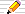icon means there is a Things to do section of questions to start your own investigations. The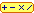calculator icon indicates that there is a live interactive calculator in that section.

1·61803 39887 49894 84820 45868 34365 63811 77203 09179 80576 ..More..## What is the golden section (or Phi)?

Also called the golden ratio or the golden mean, what is the value of the golden section?

### A simple definition of Phi

There are just two numbers that remain the same when they are squared namely 0 and 1. Other numbers get bigger and some get smaller when we square them:
Squares that are biggerSquares that are smaller
22 is 4 1/2=0·5 and 0·52 is 0·25=1/4
32 is 9 1/5=0·2 and 0·22 is 0·04=1/25
102 is 100 1/10=0·1 and 0·12 is 0·01=1/100
One definition of the golden section number is that
to square it you just add 1
or, if we let this value be denoted by the upper-case Greek letter Phi Φ in mathematics:
Phi2 = Phi + 1
In fact, there are two numbers with this property, one is Phi and another is closely related to it when we write out some of its decimal places.
Here is a mathematical derivation (or proof) of the two values. You can skip over this to the answers at the foot of this paragraph if you like.

Phi2 = Phi + 1 or, subtracting Phi + 1 from both sides:
Phi2 – Phi – 1 = 0

We can solve this quadratic equation to find two possible values for Phi as follows:

• First note that (Phi – 1/2)2 = Phi2 – Phi + 1/4
• Using this we can write Phi2 – Phi – 1 as (Phi – 1/2)25/4
and since Phi2 – Phi – 1 = 0 then (Phi – 1/2)2 must equal 5/4
• Taking square-roots gives (Phi – 1/2) = +√5/2 or –√5/2.
• so Phi = 1/2 + √5/2 or 1/2√5/2.
 The two values are : 1 + √5 = 1·6180339887... and 1 − √5 = −0·6180339887... 2 2
Did you notice that their decimal parts are identical?
The larger value 1·618... we denote using the capital Greek letter Phi written as Φ
the smaller value −0·618... is written as −φ using the small Greek letter phi.

The large P indicates the larger positive value 1·618... and the small p denotes the smaller positive value 0·618....
Φ = (√5 + 1) / 2, φ = (√5 – 1) / 2

Other names used for these values are the Golden Ratio and the Golden number. We will use the two Greek letters Phi (Φ) and phi (φ) in these pages, although some mathematicians use another Greek letters such as tau (τ) or else alpha (α) and beta (β).
Here, Phi (large P) is the larger value, 1.618033.... and phi (small p) is the smaller positive value 0.618033... which is also just Phi – 1.

As a little practice at algebra, use the expressions above to show that φ × Φ = 1.

Here is a summary of what we have found already that we will find useful in what follows:

 Phi phi = 1    Phi - phi = 1      Phi + phi = √5 Phi = 1.6180339.. phi = 0.6180339.. Phi = 1 + phi phi = Phi – 1 Phi = 1/phi phi = 1/Phi Phi2 = Phi + 1 (–phi)2 = –phi + 1 or phi2 = 1 – phi Phi = (√5 + 1)/2 phi = (√5 – 1)/2

1·61803 39887 49894 84820 45868 34365 63811 77203 09179 80576 ..More..### A bit of history...

Euclid, the Greek mathematician of about 300BC, wrote the Elements which is a collection of 13 books on Geometry (written in Greek originally). It was the most important mathematical work until this century, when Geometry began to take a lower place on school syllabuses, but it has had a major influence on mathematics.

It starts from basic definitions called axioms or "postulates" (self-evident starting points). An example is the fifth axiom that

there is only one line parallel to another line through a given point.
From these Euclid develops more results (called propositions) about geometry which he proves based purely on the axioms and previously proved propositions using logic alone. The propositions involve constructing geometric figures using a straight edge and compasses only so that we can only draw straight lines and circles.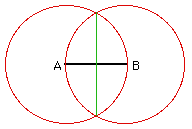For instance, Book 1, Proposition 10 to find the exact centre of any line AB
1. Put your compass point on one end of the line at point A.
2. Open the compasses to the other end of the line, B, and draw the circle.
3. Draw another circle in the same way with centre at the other end of the line.
4. This gives two points where the two red circles cross and, if we join these points, we have a (green) straight line at 90 degrees to the original line which goes through its exact centre.

In Book 6, Proposition 30, Euclid shows how to divide a line in mean and extreme ratio which we would call "finding the golden section G point on the line". He describes this geometrically.

```                <-------- 1 --------->
A            G       B
g        1–g
```
Euclid used this phrase to mean the ratio of the smaller part of this line, GB to the larger part AG (ie the ratio GB/AG) is the SAME as the ratio of the larger part, AG, to the whole line AB (i.e. is the same as the ratio AG/AB). We can see that this is indeed the golden section point if we let the line AB have unit length and AG have length g (so that GB is then just 1–g) then the definition means that
 GB = AG i.e. using the lengths of the sections 1-g = gAG AB g 1
which we rearrange to get 1 – g = g2.
Notice that earlier we defined Phi2 as Phi+1 and here we have g2 = 1–g or g2+g=1.
We can solve this in the same way that we found Phi above:
 g = −1 + √5 or g = −1 − √5 2 2

So there are two numbers which when added to their squares give 1. For our geometrical problem, g is a positive number so the first value is the one we want. This is our friend phi also equal to Phi–1 (and the other value is merely –Phi).

It seems that this ratio had been of interest to earlier Greek mathematicians, especially Pythagoras (580BC - 500BC) and his "school". There is an interesting article on The Golden ratio at the St Andrew's MacTutor History of Mathematics site.

####You do the maths...1. Suppose we labelled the parts of our line as follows:
```                 A            G       B
x          1
```
so that AB is now has length 1+x. If Euclid's "division of AB into mean and extreme ratio" still applies to point G, what quadratic equation do you now get for x? What is the value of x?

#### Links on Euclid and his "Elements"

• The Thirteen Books of Euclid's Elements, Books 1 and 2
• The Thirteen Books of Euclid's Elements, Books 3 to 9
• The Thirteen Books of Euclid's Elements, Books 10 to 13
all are by Thomas L Heath who translated and annotated Euclid's work, each is about 464 pages, published by Dover in paperback, second edition 1956.

## Phi and the Egyptian Pyramids?

The Rhind Papyrus of about 1650 BC is one of the oldest mathematical works in existence, giving methods and problems used by the ancient Babylonians and Egyptians. It includes the solution to some problems about pyramids but it does not mention anything about the golden ratio Phi.

The ratio of the length of a face of the Great Pyramid (from centre of the bottom of a face to the apex of the pyramid) to the distance from the same point to the exact centre of the pyramid's base square is about 1·6. It is a matter of debate whether this was "intended" to be the golden section number or not.
According to Elmer Robinson (see the reference below), using the average of eight sets of data, says that "the theory that the perimeter of the pyramid divided by twice its vertical height is the value of pi" fits the data much better than the theory above about Phi.
The following references will explain circumstantial evidence for and against:

• How to Find the "Golden Number" without really trying Roger Fischler, Fibonacci Quarterly, 1981, Vol 19, pp 406 - 410
Case studies include the Great Pyramid of Cheops and the various theories propounded to explain its dimensions, the golden section in architecture, its use by Le Corbusier and Seurat and in the visual arts. He concludes that several of the works that purport to show Phi was used are, in fact, fallacious and "without any foundation whatever".
• The Fibonacci Drawing Board Design of the Great Pyramid of Gizeh Col. R S Beard in Fibonacci Quarterly vol 6, 1968, pages 85 - 87;
has three separate theories (only one of which involves the golden section) which agree quite well with the dimensions as measured in 1880.
• A Note on the Geometry of the Great Pyramid Elmer D Robinson in The Fibonacci Quarterly vol 20 (1982) page 343
shows that the theory involving pi fits much better than the one regarding Phi.
• George Markowsky's Misconceptions about the Golden ratio in The College Mathematics Journal Vol 23, January 1992, pages 2-19.
This is readable and well presented. You may or may not agree with all that Markowsky says, but this is a very good article that tries to debunk a simplistic and unscientific "cult" status being attached to Phi, seeing it where it really is not! He has some convincing arguments that Phi does not occur in the measurements of the Egyptian pyramids.

## Other names for Phi

Euclid (about 300BC) in his "Elements" calls dividing a line at the 0.6180399.. point dividing a line in the extreme and mean ratio. This later gave rise to the name golden mean.

There are no extant records of the Greek architects' plans for their most famous temples and buildings (such as the Parthenon). So we do not know if they deliberately used the golden section in their architectural plans. The American mathematician Mark Barr used the Greek letter phi (φ) to represent the golden ratio, using the initial letter of the Greek Phidias who used the golden ratio in his sculptures.

Luca Pacioli (sometimes written as Paccioli), 1445-1517, wrote a book called De Divina Proportione (The Divine Proportion) in 1509. It contains drawings made by Leonardo da Vinci of the 5 Platonic solids. It was probably Leonardo (da Vinci) who first called it the sectio aurea (Latin for the golden section).

Today, some mathematicians use phi (φ) for the golden ratio as on these web pages and others use the Greek letters alpha (α) or tau (τ), the initial letter of tome which is the Greek work for "cut".

1·61803 39887 49894 84820 45868 34365 63811 77203 09179 80576 ..More..## The Value of Phi

### Phi to 2000 decimal places

 Φ has the value √5 + 1 and φ is √5 – 1 .2 2

Both have identical fractional parts after the decimal point. Both are also irrational which means that
• They cannot be written as M/N for any whole numbers M and N;
• their decimal fraction parts have no pattern in their digits, that is, they never end up repeating a fixed cycle of digits
Later on this page we will show why Φ and φ cannot be written as exact fractions. There is another surprise in store later when we find which fractions are the best approximations to Φ.

Here is the decimal value of Φ to 2000 places grouped in blocks of 5 decimal digits. The value of φ is identical but begins with 0·6.. instead of 1·6.. .
Read this as ordinary text, in lines across, so Φ is 1·61803398874...)

 ``` Dps: 1·61803 39887 49894 84820 45868 34365 63811 77203 09179 80576 50 28621 35448 62270 52604 62818 90244 97072 07204 18939 11374 100 84754 08807 53868 91752 12663 38622 23536 93179 31800 60766 72635 44333 89086 59593 95829 05638 32266 13199 28290 26788 200 06752 08766 89250 17116 96207 03222 10432 16269 54862 62963 13614 43814 97587 01220 34080 58879 54454 74924 61856 95364 300 86444 92410 44320 77134 49470 49565 84678 85098 74339 44221 25448 77066 47809 15884 60749 98871 24007 65217 05751 79788 400 34166 25624 94075 89069 70400 02812 10427 62177 11177 78053 15317 14101 17046 66599 14669 79873 17613 56006 70874 80710 500 13179 52368 94275 21948 43530 56783 00228 78569 97829 77834 78458 78228 91109 76250 03026 96156 17002 50464 33824 37764 86102 83831 26833 03724 29267 52631 16533 92473 16711 12115 88186 38513 31620 38400 52221 65791 28667 52946 54906 81131 71599 34323 59734 94985 09040 94762 13222 98101 72610 70596 11645 62990 98162 90555 20852 47903 52406 02017 27997 47175 34277 75927 78625 61943 20827 50513 12181 56285 51222 48093 94712 34145 17022 37358 05772 78616 00868 83829 52304 59264 78780 17889 92199 02707 76903 89532 19681 98615 14378 03149 97411 06926 08867 42962 26757 56052 31727 77520 35361 39362 1000 10767 38937 64556 06060 59216 58946 67595 51900 40055 59089 50229 53094 23124 82355 21221 24154 44006 47034 05657 34797 66397 23949 49946 58457 88730 39623 09037 50339 93856 21024 23690 25138 68041 45779 95698 12244 57471 78034 17312 64532 20416 39723 21340 44449 48730 23154 17676 89375 21030 68737 88034 41700 93954 40962 79558 98678 72320 95124 26893 55730 97045 09595 68440 17555 19881 92180 20640 52905 51893 49475 92600 73485 22821 01088 19464 45442 22318 89131 92946 89622 00230 14437 70269 92300 78030 85261 18075 45192 88770 50210 96842 49362 71359 25187 60777 88466 58361 50238 91349 33331 22310 53392 32136 24319 26372 89106 70503 39928 22652 63556 20902 97986 42472 75977 25655 08615 48754 35748 26471 81414 51270 00602 38901 62077 73224 49943 53088 99909 50168 03281 12194 32048 19643 87675 86331 47985 71911 39781 53978 07476 15077 22117 50826 94586 39320 45652 09896 98555 67814 10696 83728 84058 74610 33781 05444 39094 36835 83581 38113 11689 93855 57697 54841 49144 53415 09129 54070 05019 47754 86163 07542 26417 29394 68036 73198 05861 83391 83285 99130 39607 20144 55950 44977 92120 76124 78564 59161 60837 05949 87860 06970 18940 98864 00764 43617 09334 17270 91914 33650 13715 2000 ```

### Phi to 1,500,000,000 places!

Simon Plouffe of Simon Fraser University notes that Greg J Fee programmed a method of his to compute the golden ratio (Phi) to ten million places in December 1996. He used Maple and it took 29 minutes and 16 seconds on an SGI R10000 194MHz computer. He lists the first 15,000 places.
The latest record is 1,000,000,000,000 places in 2010 by A.J. Yee.

### Phi in Binary

Using base 2 (binary), is there a pattern in the digits of Phi? Let's have a look at the first 500 binary-digits (or bits):

 ```1·10011 11000 11011 10111 10011 01110 01011 11111 01001 01001 11110 00001 01011 11100 11100 11100 11000 00001 10000 00101 100 11001 11011 01110 01000 00110 10000 01000 01000 00100 01001 11011 01011 11110 01110 10001 00111 00100 10100 01111 11000 200 01101 10001 10101 00001 00011 10100 00110 00001 10001 11010 01010 10010 01110 11001 11111 10000 10110 00101 01001 11101 300 00100 11110 11011 11111 00000 01101 00011 10000 01000 10110 11010 11011 11110 00110 00001 00111 11110 00000 01100 01000 400 01101 11100 00100 10010 10000 10000 00001 10000 00000 01011 00000 11101 01100 10010 11101 00100 00001 11100 11001 10101 500 ```
Here is a text file of 25,000 binary places (opens in a new window, 28K).

Neither the decimal form of Phi, nor the binary one nor any other base have any ultimate repeating pattern in their digits.
This is because Phi is not a fration, an ir-rational number, and so its "decimal fraction" never gets into a repeating pattern in any base. Fractions always stop or else end up repeating the same pattern in their "decimal fraction".
For more on this, the patterns and why any fraction either stops or repeats and much more see Fractions and Decimals.

1·61803 39887 49894 84820 45868 34365 63811 77203 09179 80576 ..More..## Phi and the Fibonacci numbers

On the Fibonacci and Nature page we saw a graph which showed that the ratio of successive Fibonacci numbers gets closer and closer to Φ.

Here is the connection the other way round, where we can discover the Fibonacci numbers arising from the number Φ.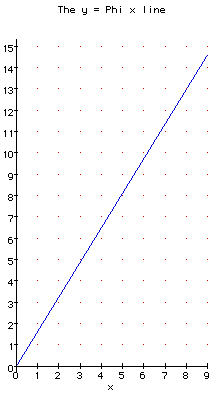The graph on the right shows a line whose gradient is Φ, that is the line

y = Φ x = 1·6180339.. x

Since Φ is not the ratio of any two integers, the graph will never go through any points of the form (i,j) where i and j are whole numbers - apart from one trivial exception - can you spot it?
What are the nearest integer-coordinate points to the Φ line?
Let's start at the origin and work up the line.
The first is (0,0) of course, so these are the two integer coordinates of the only whole-number point exactly on the line! In fact ANY line y = k x will go through the origin, so that is why we will ignore this point as a "trivial exception" (as mathematicians like to put it).
The next point close to the line looks like (0,1) although (1,2) is nearer still. The next nearest seems even closer: (2,3) and (3,5) is even closer again. So far our sequence of "integer coordinate points close to the Phi line" is as follows: (0,1), (1,2), (2,3), (3,5)
What is the next closest point? and the next? Surprised? The coordinates are successive Fibonacci numbers!

Let's call these the Fibonacci points. Notice that the ratio y/x for each Fibonacci point (x,y) gets closer and closer to Phi = 1·618... but the interesting point that we see on this graph is that

the Fibonacci points are the closest points to the Φ line.

1·61803 39887 49894 84820 45868 34365 63811 77203 09179 80576 ..More..### The Ratio of neighbouring Fibonacci Numbers tends to Phi

On the Fibonacci Numbers and Nature page we saw that the ratio of two neighbouring Fibonacci numbers soon settled down to a particular value near 1·6: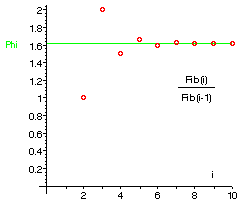In fact, the exact value is Φ.
As we take larger and larger Fibonacci numbers, so their ratio gets closer and closer to Phi. Why? Here we show how this happens.

The basic Fibonacci relationship is

 F(i+2) = F(i+1) + F(i)       The Fibonacci relationship

The graph shows that the ratio F(i+1)/F(i) seems to get closer and closer to a particular value, which for now we will call X.
If we take three neighbouring Fibonacci numbers, F(i), F(i+1) and F(i+2) then, for very large values of i, the ratio of F(i) and F(i+1) will be almost the same as the ratio of F(i+1) and F(i+2), so let's see what happens if both of these are the same value: X.

 F(i+1) = F(i+2) = XF(i) F(i+1)
(A)
But, using the The Fibonacci relationship we can replace F(i+2) by F(i+1) + F(i) and then simplify the resulting fraction, as follows:
 F(i+2)F(i+1)
=
 F(i+1) + F(i)F(i+1)
=
 F(i+1) + F(i)F(i+1) F(i+1)
=
 1 + F(i)F(i+1)
(B)
So, replacing F(i+2)/F(i+1) in (A) by the right hand side of (B), we have:
 X = F(i+1) = 1 + F(i)F(i) F(i+1)
But the last fraction is F(i)/F(i+1) and is the reciprocal of F(i+1)/F(i) which, from (A) is just X.
So we can replace F(i)/F(i+1) by 1/X and we then have an equation purely in terms of X:
 X = F(i+1) = 1 + F(i) = 1 + 1F(i) F(i+1) X
Multiplying both sides by X gives:
 X = 1 + 1X
X2 = X + 1
But we have seen this equation before in A simple definition of Phi so know that X is, indeed, exactly Φ!

Remember, this supposed that the ratio of two pairs of neighbours in the Fibonacci series was the same value. This only happens "in the limit" as mathematicians say. So what happens is that, as the series progresses, the ratios get closer and closer to this limiting value, or, in other words, the ratios get closer and closer to Φ the further down the series that we go.

Did you notice that we have not used the two starting values in this proof? No matter what two values we start with, if we apply the Fibonacci relationship to continue the series, the ratio of two terms will (in the limit) always be Phi!

#### But there are two values that satisfy X2 = X + 1 aren't there?

Yes, there are. The other value, –phi which is –0·618... is revealed if we extend the Fibonacci series backwards. We still maintain the same Fibonacci relationship but we can find numbers before 0 and still keep this relationship:
 i Fib(i) ... –10 –9 –8 –7 –6 –5 –4 –3 –2 –1 0 1 2 3 4 5 6 7 8 9 10 ... ... –55 34 –21 13 –8 5 –3 2 –1 1 0 1 1 2 3 5 8 13 21 34 55 ...
When we use this complete Fibonacci series and plot the ratios F(i)/F(i–1) we see that the ratios on the left-hand side of 0 are
 1 = –1, –1 = –0.5, 2 = –0.666.., –3 = –0.6, 5 = –0.625, ...–1 2 –3 5 –8
Plotting these shows both solutions to X2 = X + 1:-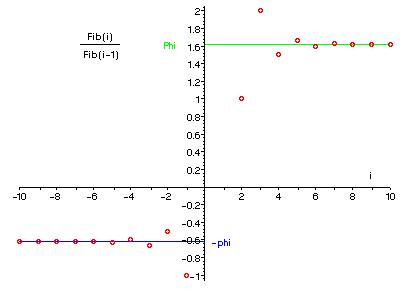### More on Fibonacci Number ratios

What if we take the ratio not of neighbouring Fibonacci numbers but Fibonacci numbers one apart, i.e. F(n)/F(n-2)?

Experiment with a spreadsheet (or calculator) and you will soon find that F(n)/F(n-2) gets closer and closer to 2.618... as n gets larger. This is Φ+1 and also Φ2.

What if the numbers are 3 apart i.e. F(n)/F(n-3)?
This time the ratio gets closer to 4.236... or Φ3
Is there a general rule for the limit of F(n)/F(n-k)?
Yes! This ratio gets closer and closer to Φk.

If is easy to verify this using a small variation on the method above (where we showed F(i)/F(i+1) gets closer to Φ as i gets larger), or using Binet's Formula that we met earlier on the page called A Formula For Fib(n).

## Another definition of Phi

We defined Φ to be (one of the two values given by)
Phi2 = Phi+1

Suppose we divide both sides of this equation by Phi:
Phi = 1 + 1/Phi

Here is another definition of Φ - that number which is 1 more than its reciprocal
(the reciprocal of a number is 1 over it so that, for example, the reciprocal of 2 is 1/2 and the reciprocal of 9 is 1/9).

## Phi as a continued fraction

Look again at the last equation:
Phi = 1 + 1/Phi
This means that wherever we see Phi (Φ) we can substitute (1 + 1/Phi).
Since we see Phi on the right hand side, lets substitute it in there!
Phi = 1 + 1/(1 + 1/Phi)
In fact, we can do this again and again and get:
 Phi = 1 + 1 1 + 1 1 + 1 1 + ..
This unusual expression is called a continued fraction since we continue to form fractions underneath fractions underneath fractions.

This continued fraction has a big surprise in store for us....

### Phi is not a fraction

But Phi is a fraction .. it is (√5 + 1)/2.
Here, by a fraction we mean a number fraction such as 2/3 or 17/24 or 12/7. The first is a proper fraction since it are less than 1. Also 5.61 is a fraction, a decimal fraction since it is 561/100, the ratio of a whole number and a power of ten.
Strictly, all whole numbers can be written as fractions if we make the denominator (the part below the line) equal to 1! However, we commonly use the word fraction when there really is a fraction in the value.

Mathematicians call all these fractional (and whole) numbers rational numbers because they are the ratio of two whole numbers and it is these number fractions that we will mean by fraction in this section.

It may seem as if all number can be written as fractions - but this is, in fact, false. There are numbers which are not the ratio of any two whole numbers, e.g. √2 = 1.41421356... , π = 3.14159..., e = 2.71828... . Such values are called ir-ratio-nal since they cannot be represented as a ratio of two whole numbers (i.e. a fraction). A simple consequence of this is that their decimal fraction expansions go on for ever and never repeat at any stage!
Any and every fraction has a decimal fraction expansion that either

• stops as in, for example, 1/8 = 0.125 exactly or else
• eventually gets into a repeating pattern that repeats for ever e.g. 5/12 = 0.416666666... , 3/7 = 0.428571 428571 428571 ...

Can we write Phi as a fraction?
The answer is "No!" and there is a surprisingly simple proof of this. Here it is. [with thanks to Prof Shigeki Matsumoto of Konan University, Japan]
First we suppose that Φ can be written as a fraction and then show this leads to a contradiction, so we are forced to the conclusion Φ cannot be written as a fraction:
Suppose Φ = a/b and that this fraction is in its lowest terms which means that:
• a and b are whole numbers
• a and b are the smallest whole numbers to represent the fraction for Phi
• Since Φ > 1 then a > b
But we know from the alternative definitions of Φ and φ that
Φ = 1/φ and φ = Φ – 1 which we put in the fraction for Φ:
Φ = 1/(Φ –1).
Now we substitute a/b for Φ:
a/b = 1/(a/b –1) = b/(ab)
But here we have another fraction for Φ that has a smaller numerator, b since a > b
which is a contradiction because we said we had chosen a and b were chosen to be the smallest whole numbers.
So we have a logical impossibility if we assume Φ can be written as a proper fraction
and the only possibility that logic allows is that Φ cannot be written as a proper fraction - Φ is irrational.

### Rational Approximations to Phi

If no fraction can be the exact value of Φ, what fractions are good approximations to Φ?

If we stop the continued fraction for Φ at various points, we get values which approximate to Φ:

 Φ = 1Φ = 1 + 11
 = 2Φ = 1 + 11 + 11
 = 32
 Φ = 1 + 11 + 11 + 11
 = 53

The next approximation is always 1 + 1/(the previous approximation) shown in green.
Did you notice that this series of fractions is just the ratios of successive Fibonacci numbers - surprise!

The proper mathematical term for these fractions which are formed from stopping a continued fraction for Φ at various points is the convergents to Φ. The series of convergents is

 1 , 2 , 3 , 5 , 8 , 13 , 21 ,   ...1 1 2 3 5 8 13

### Why do Fibonacci numbers occur in the convergents?

This is an optional section where we show exactly why the Fibonacci numbers appear in the successive approximations (the convergents) above. Skip to the next section if you like

where F(n) represents the n-th Fibonacci number.

To get from one fraction to the next, we saw that we just take the reciprocal of the fraction and add 1:
so the next one after F(1)/F(0) is
1 +
 1 F(1) / F(0)
= 1 +
 F(0) F(1)
=
 F(1) + F(0) F(1)

But the Fibonacci numbers have the property that two successive numbers add to give the next, so F(1) + F(0) = F(2) and our next fraction can be written as
 F(1) + F(0) F(1)
=
 F(2) F(1)

So starting with the ratio of the first two Fibonacci numbers the next convergent to Φ is the ratio of the next two Fibonacci numbers.

This always happens:
if we have F(n)/F(n – 1) as a convergent to Φ, then the next convergent is F(n+1)/F(n).

We will get all the ratios of successive Fibonacci numbers as values which get closer and closer to Φ.

You can find out more about continued fractions and how they relate to splitting a rectangle into squares and also to Euclid's algorithm on the Introduction to Continued Fractions page at this site.

1·61803 39887 49894 84820 45868 34365 63811 77203 09179 80576 ..More..## Other ways to find Phi using your calculator

Here are two more interesting ways to find it.

### Calculator Method 1: Invert and Add 1

• Enter 1 to start the process.
• Take its reciprocal (the 1/x button or else xy and power y:-1). Add 1.
• Take its reciprocal. Add 1.
• Take its reciprocal. Add 1.
• Keep repeating the last step until the display is constant.
Keep repeating these two operations (take the reciprocal, add 1) and you will find that soon the display does not alter and settles down ("converging" as mathematicians call it) to a particular value, namely 1.61803... .

In fact, you can start with many values but not all (for instance 0 or -1 will cause problems) and it will still converge to the same value: Phi.

#### Why?

The formula Φ = 1 + 1/Φ shows us where the two instructions come from.
To start, we note that the simplest approximation to the continued fraction above is just 1.

####You do the maths...1. In Calculator method 1, 0 causes a problem because we cannot take its reciprocal.
So if x is -1, when we take its reciprocal (1/-1 = -1 ) and add 1 we get 0. So 0 and -1 are bad choices since they don't lead to Phi.
What value of x will give -1? And what value of x would give that value?
Can you find a whole series of numbers which, in fact, do not lead to Phi with Calculator method 1?
[Thanks to Warren Criswell for this problem.]

####Method 1 Calculator: + and /

+ and / C A L C U L A T O R
x=

R E S U L T S

### Calculator Method 2: Add 1 and take the square-root

Here is another way to get Φ on your calculator.
• Enter any number (whole or fractional) but it must be bigger than –1.
• Add 1. Take its square root.
• Add 1. Take its square root.
• Keep repeating the last step until the display is constant
Keep repeating these two instructions and you will find it too converges to Φ.

#### Why?

This time we have used the other definition of Phi, namely
Φ2 = Φ + 1
or, taking the square root of both sides:
Φ = √Φ + 1
Can you see why we must start with a number which is not smaller (i.e. is not more negative) than –1?

####Method 2 Calculator: + and √

C A L C U L A T O Rx=

R E S U L T S

If one value tends towards Phi, what about the other value (the result after pressing the "x+1" button)?

1·61803 39887 49894 84820 45868 34365 63811 77203 09179 80576 ..More..## Similar numbers

Robert Kerr Baxter wrote to me about other numbers that have the Φ property that when you square them their decimal parts remain the same:
Phi = 1.618033.. and Phi2 is 2.618033..
 Φ has the value √5 + 1 2
Rob had noticed that this happens if we replace the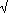5 with13 or17 or21 and so on. The series of number here is 5, (9), 13, 17, 21, (25), 29, ... which are the numbers that are 1 more than the multiples of 4. The numbers 9 and 25 are in brackets because they are perfect squares, so taking their square roots gives a whole number - in fact, an odd number - so when we add 1 and divide the result by two we just get a whole number with .00000... as the decimal part.

### Why does this happen?

Algebra can come to our help here and it is a nice application of "Solving Quadratics" that we have already seen in the first section on this page.

We want to find a formula for the numbers (x, say) "that have the same decimal part as their squares". So, if we subtract x from x2, the result will be a whole number because the decimal parts were identical.
Let's call this difference N, remembering that it is a whole number.
So

the difference between x2 and x is N, a whole number
is a description of these numbers in words. We can write this in the language of mathematics as follows:
x2 – x = Nor, adding x to both sides:x2 = N + x
and we can "solve" it in exactly the same way as we did for Φ's quadratic: x2 = 1 + x. The formula for x this time is
 x = 1 ± √1 + 4 N2
You can see that, under the square-root sign, we have 1 plus a multiple of 4 which gives the series:
 N: 1+4N: 1 2 3 4 5 ... 5 9 13 17 21 ...

For example: if we choose N = 5, then the number x (that increases by exactly 5 when squared) is

 x = 1 ± √1 + 4×5 = 1 ±21 = 2.791287847.. and x2 = 7.791287847... = 5 + x22
Checking we see that the square of this x is exactly N = 5 more than the original number x.

Another example: take Φ, which is (1 + √5)/2 or (1 + √(1+4×1))/2 so that N = 1.
Thus we can "predict" that Φ squared will be >1 more than Φ itself and, indeed, Phi = 1.618033.. and Phi2 = 2.618033.. .
We can do the same for other whole number values for N.

More generally: There is nothing in the maths of this section that prevents N from being any number, for instance 0·5 or π. Suppose N is &pi = 3.1415926535... . We can find the number x that, when squared, increases by exactly π! It is

 x = 1 ± √1 + 4 π = 1 ± √12.566370614... = 2·3416277185...22
and x2 = 5·483220372... = 2·3416277185... + 3.1415926535...

####+ / √ Calculator

+ / √ C A L C U L A T O Rx=

####You do the maths...1. Make a table of the first few numbers similar to Phi in this way, starting with Phi and its square.
2. We have only used the + sign in the formula for x above, giving positive values of x.
What negative values of x are there, that is negative numbers which, when squared (becoming positive) have exactly the same decimal fraction part?
3. What is the number that can be squared by just adding 0·5?
4. Is there an upper limit to the size of N?
Can you use the formula to find two numbers that increase by one million (1,000,000) when squared?
5. Can N be negative?
6. For instance, can we use the formula to find a number (as we have seen, there are two of them) that is 0·5 smaller when it is squared?
7. What about a number that decreases by 1 when it is squared?
8. Is there a lower limit for the value of N?
We look at some other numbers similar to Phi but in a different way on the (optional) Continued Fractions page. This time we find numbers which are like the Golden Mean, Phi, in that their decimal fraction parts are the same when we take their reciprocals, i.e. find 1/x. They are called the Silver Means.© 1996-2016 Dr Ron Knott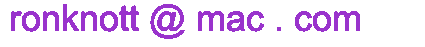updated 25 Septembr 2016